# Hypothesis Testing

Computer Simulation using examples from `infer` package. Code for quiz 13

Load the R packages that we will use

``````library(tidyverse)
library(infer)
library(skimr)
``````

# Question: t-test

• Set random seed generator to 123

• Load data into R, assign it to `hr`

``````set.seed(123)

col_types = "fddfff")
``````

• Use `skim` to summarize the data in `hr`
``````skim(hr)
``````
 Name hr Number of rows 500 Number of columns 6 _______________________ Column type frequency: factor 4 numeric 2 ________________________ Group variables None

Variable type: factor

skim_variable n_missing complete_rate ordered n_unique top_counts
gender 0 1 FALSE 2 mal: 256, fem: 244
evaluation 0 1 FALSE 4 bad: 154, fai: 142, goo: 108, ver: 96
salary 0 1 FALSE 6 lev: 95, lev: 94, lev: 87, lev: 85
status 0 1 FALSE 3 fir: 194, pro: 179, ok: 127

Variable type: numeric

skim_variable n_missing complete_rate mean sd p0 p25 p50 p75 p100 hist
age 0 1 39.86 11.55 20.3 29.60 40.2 50.1 59.9 ▇▇▇▇▇
hours 0 1 49.39 13.15 35.0 37.48 45.6 58.9 79.9 ▇▃▂▂▂
• The mean hours worked per week is 49.4.

Is the mean number of hours worked per week 48?

• `specify` that `hours` is the variable of interest.
``````hr %>%
specify(response = hours)
``````
``````Response: hours (numeric)
# A tibble: 500 x 1
hours
<dbl>
1  78.1
2  35.1
3  36.9
4  38.5
5  36.1
6  78.1
7  76
8  35.6
9  35.6
10  56.8
# ... with 490 more rows``````

• `hypothesize` that the average hours worked is 48
``````hr %>%
specify(response = hours) %>%
hypothesize(null = "point", mu = 48)
``````
``````Response: hours (numeric)
Null Hypothesis: point
# A tibble: 500 x 1
hours
<dbl>
1  78.1
2  35.1
3  36.9
4  38.5
5  36.1
6  78.1
7  76
8  35.6
9  35.6
10  56.8
# ... with 490 more rows``````

• `generate` 1000 replicates representing the null hypothesis
``````hr %>%
specify(response = hours) %>%
hypothesize(null = "point", mu = 48) %>%
generate(reps = 1000, type = "bootstrap")
``````
``````Response: hours (numeric)
Null Hypothesis: point
# A tibble: 500,000 x 2
# Groups:   replicate [1,000]
replicate hours
<int> <dbl>
1         1  39.7
2         1  44.3
3         1  46.8
4         1  33.7
5         1  39.6
6         1  39.5
7         1  40.5
8         1  55.8
9         1  72.6
10         1  35.7
# ... with 499,990 more rows``````
• The output has 500,000 rows

• `calculate` the distribution of statistics from the generated data

• assign output to `null_t_distribution`

• display `null_t_distribution`

``````null_t_distribution <- hr %>%
specify(response = age) %>%
hypothesize(null = "point", mu = 48) %>%
generate(reps = 1000, type = "bootstrap") %>%
calculate(stat = "t")

null_t_distribution
``````
``````Response: age (numeric)
Null Hypothesis: point
# A tibble: 1,000 x 2
replicate     stat
<int>    <dbl>
1         1  0.144
2         2 -1.72
3         3  0.404
4         4 -1.11
5         5  0.00894
6         6  1.46
7         7 -0.905
8         8 -0.663
9         9  0.291
10        10  3.09
# ... with 990 more rows``````
• `null_t_distribution` has 1000 t-stats.

• `visualize` the simulated null distribution.
``````visualise(null_t_distribution)
``````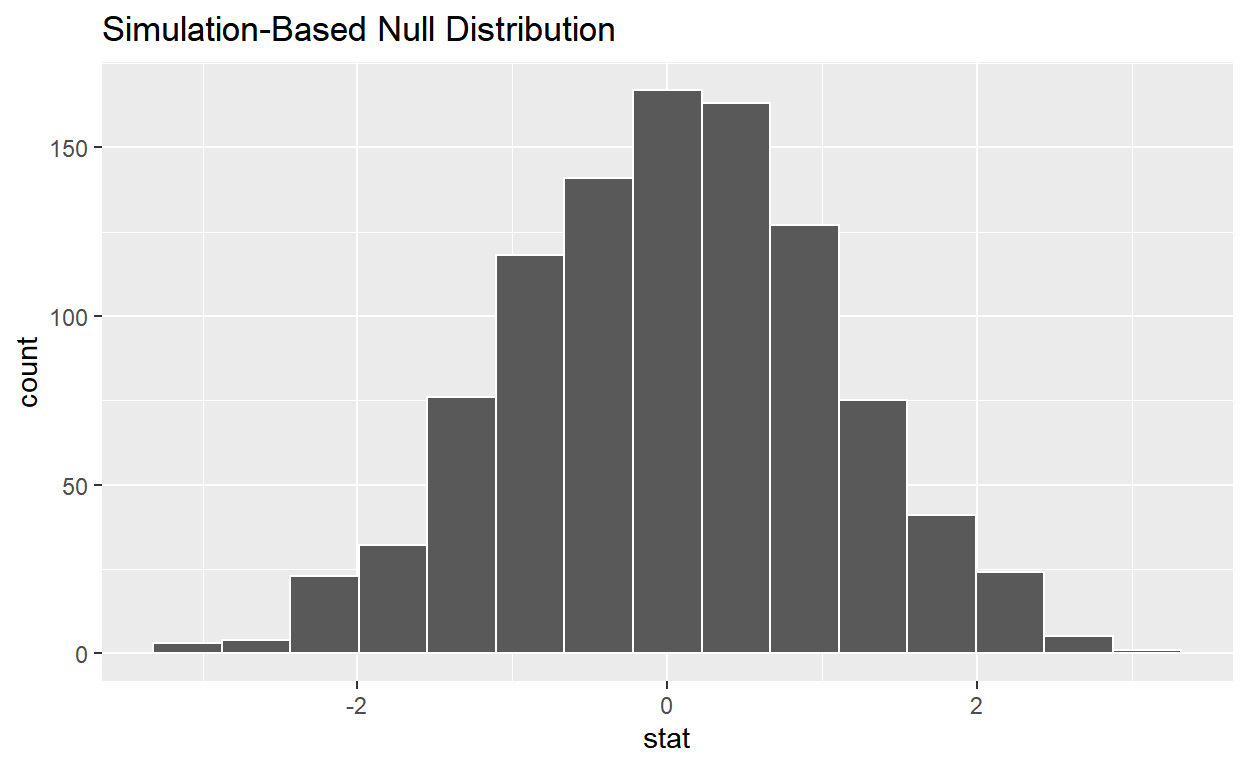• `calculate` the statistic from your observed data.

• assign the output to `observed_t_statistic`

• display `observed_t_statistic`

``````observed_t_statistic <- hr %>%
specify(response = hours) %>%
hypothesize(null = "point", mu = 48) %>%
calculate(stat = "t")

observed_t_statistic
``````
``````Response: hours (numeric)
Null Hypothesis: point
# A tibble: 1 x 1
stat
<dbl>
1  2.37``````

• `get_p_value` from the simulated null distribution and the observed statistic.
``````null_t_distribution %>%
get_p_value(obs_stat = observed_t_statistic, direction = "two-sided")
``````
``````# A tibble: 1 x 1
p_value
<dbl>
1   0.014``````

• `shade_p_value` on the simulated null distribution.
``````null_t_distribution %>%
visualize() +
shade_p_value(obs_stat = observed_t_statistic, direction = "two-sided")
``````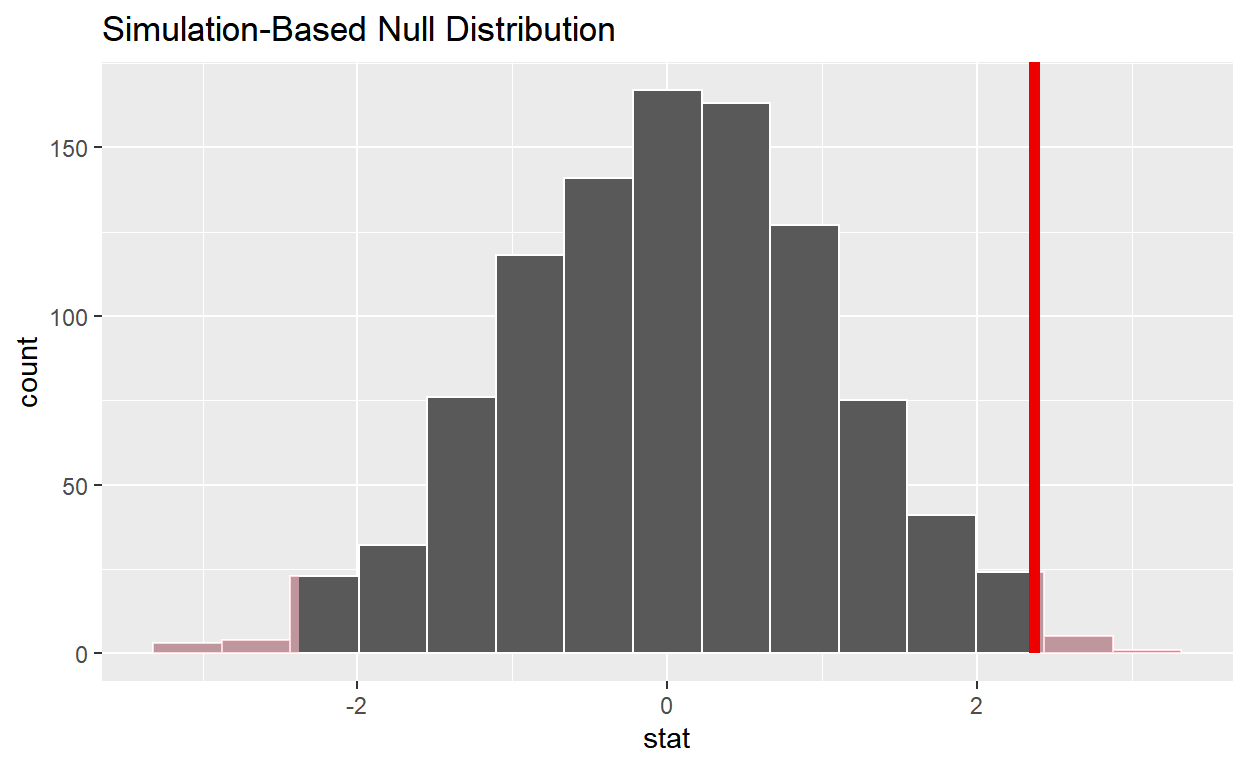• Is the p-value < 0.05? `yes`

• Does your analysis support the null hypothesis that the true mean number of hours worked was 48? `no`

# Question: Sample t-test 2

• Load data into R, assign it to `hr_2`
``````hr_2 <- read_csv("https://estanny.com/static/week13/data/hr_1_tidy.csv",
col_types = "fddfff")
``````

Is the average number of hours worked the same for both genders in hr_2?

• Use `skim` to summarize the data in `hr_2` by gender.
``````hr_2 %>%
group_by(gender) %>%
skim()
``````
 Name Piped data Number of rows 500 Number of columns 6 _______________________ Column type frequency: factor 3 numeric 2 ________________________ Group variables gender

Variable type: factor

skim_variable gender n_missing complete_rate ordered n_unique top_counts
evaluation female 0 1 FALSE 4 fai: 81, bad: 71, ver: 57, goo: 51
evaluation male 0 1 FALSE 4 bad: 82, fai: 61, goo: 55, ver: 42
salary female 0 1 FALSE 6 lev: 54, lev: 50, lev: 44, lev: 41
salary male 0 1 FALSE 6 lev: 52, lev: 47, lev: 46, lev: 39
status female 0 1 FALSE 3 fir: 96, pro: 87, ok: 77
status male 0 1 FALSE 3 fir: 89, ok: 76, pro: 75

Variable type: numeric

skim_variable gender n_missing complete_rate mean sd p0 p25 p50 p75 p100 hist
age female 0 1 41.78 11.50 20.5 32.15 42.35 51.62 59.9 ▆▅▇▆▇
age male 0 1 39.32 11.55 20.2 28.70 38.55 49.52 59.7 ▇▇▆▇▆
hours female 0 1 50.32 13.23 35.0 38.38 47.80 60.40 79.7 ▇▃▃▂▂
hours male 0 1 48.24 12.95 35.0 37.00 42.40 57.00 78.1 ▇▂▂▁▂
• Females worked an average of 50.3 hours per week.

• Males worked an average of 48.2 hours per week.

• Use `geom_boxplot` to plot distribution of hours worked by gender.
``````hr_2 %>%
ggplot(aes(x = gender, y = hours)) +
geom_boxplot()
``````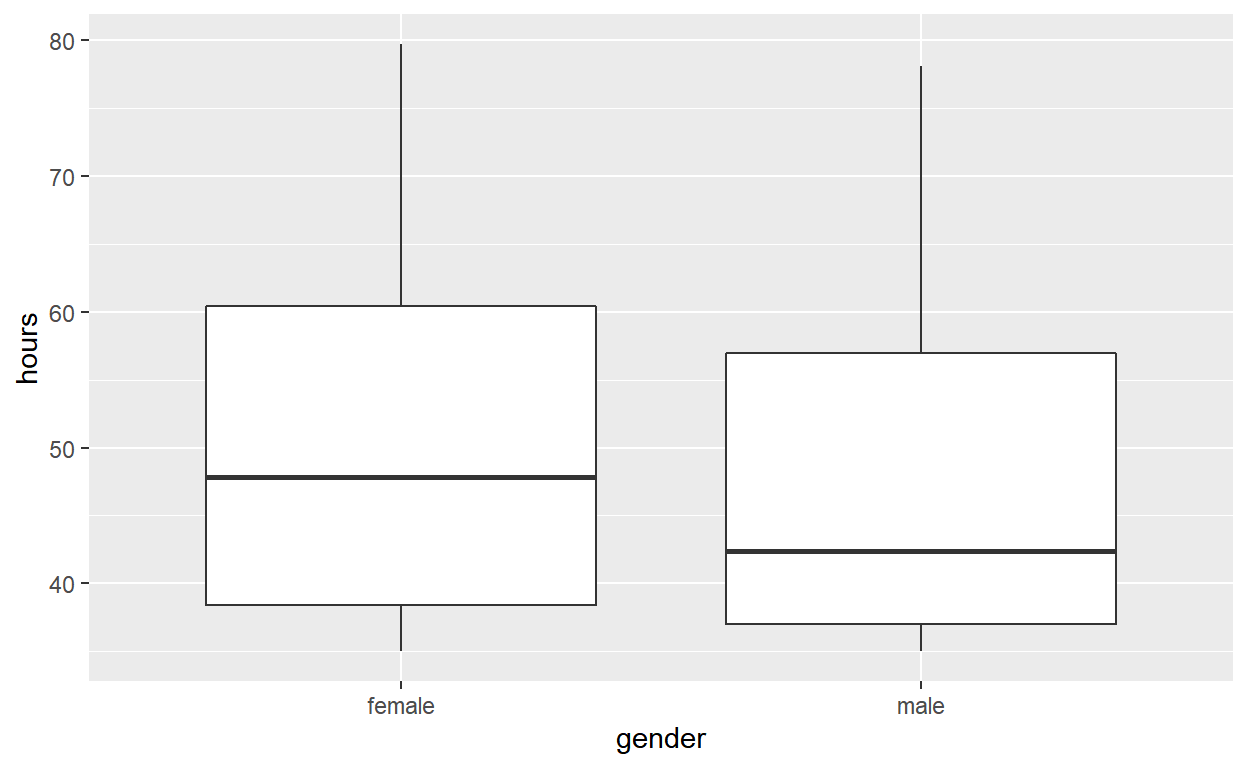• `specify` the variables of interest are hours and gender
``````hr_2 %>%
specify(response = hours, explanatory = gender)
``````
``````Response: hours (numeric)
Explanatory: gender (factor)
# A tibble: 500 x 2
hours gender
<dbl> <fct>
1  36.5 female
2  55.8 female
3  35   male
4  52   female
5  35.1 male
6  36.3 female
7  40.1 female
8  42.7 female
9  66.6 male
10  35.5 male
# ... with 490 more rows``````

• `hypothesize` that the number of hours worked and gender are independent.
``````hr_2 %>%
specify(response = hours, explanatory = gender) %>%
hypothesize(null = "independence")
``````
``````Response: hours (numeric)
Explanatory: gender (factor)
Null Hypothesis: independence
# A tibble: 500 x 2
hours gender
<dbl> <fct>
1  36.5 female
2  55.8 female
3  35   male
4  52   female
5  35.1 male
6  36.3 female
7  40.1 female
8  42.7 female
9  66.6 male
10  35.5 male
# ... with 490 more rows``````

• `generate` 1000 replicates representing the null hypothesis
``````hr_2 %>%
specify(response = hours, explanatory = gender) %>%
hypothesize(null = "independence") %>%
generate(reps = 1000, type = "permute")
``````
``````Response: hours (numeric)
Explanatory: gender (factor)
Null Hypothesis: independence
# A tibble: 500,000 x 3
# Groups:   replicate [1,000]
hours gender replicate
<dbl> <fct>      <int>
1  36.4 female         1
2  35.8 female         1
3  35.6 male           1
4  39.6 female         1
5  35.8 male           1
6  55.8 female         1
7  63.8 female         1
8  40.3 female         1
9  56.5 male           1
10  50.1 male           1
# ... with 499,990 more rows``````
• The output has 500,000 rows.

• `calculate` the distribution of statistics from the generated data.

• Assign output to `null_distribution_2_sample_permute`

• Display `null_distribution_2_sample_permute`

``````null_distribution_2_sample_permute <- hr_2 %>%
specify(response = hours, explanatory = gender) %>%
hypothesize(null = "independence") %>%
generate(reps = 1000, type = "permute") %>%
calculate(stat = "t", order = c("female", "male"))

null_distribution_2_sample_permute
``````
``````Response: hours (numeric)
Explanatory: gender (factor)
Null Hypothesis: independence
# A tibble: 1,000 x 2
replicate   stat
<int>  <dbl>
1         1 -0.208
2         2 -0.328
3         3 -2.28
4         4  0.528
5         5  1.60
6         6  0.795
7         7  1.24
8         8 -3.31
9         9  0.517
10        10  0.949
# ... with 990 more rows``````
• `null_t_distribution` has 1000 t-stats.

• `visualize` the simulated null distribution.
``````visualize(null_distribution_2_sample_permute)
``````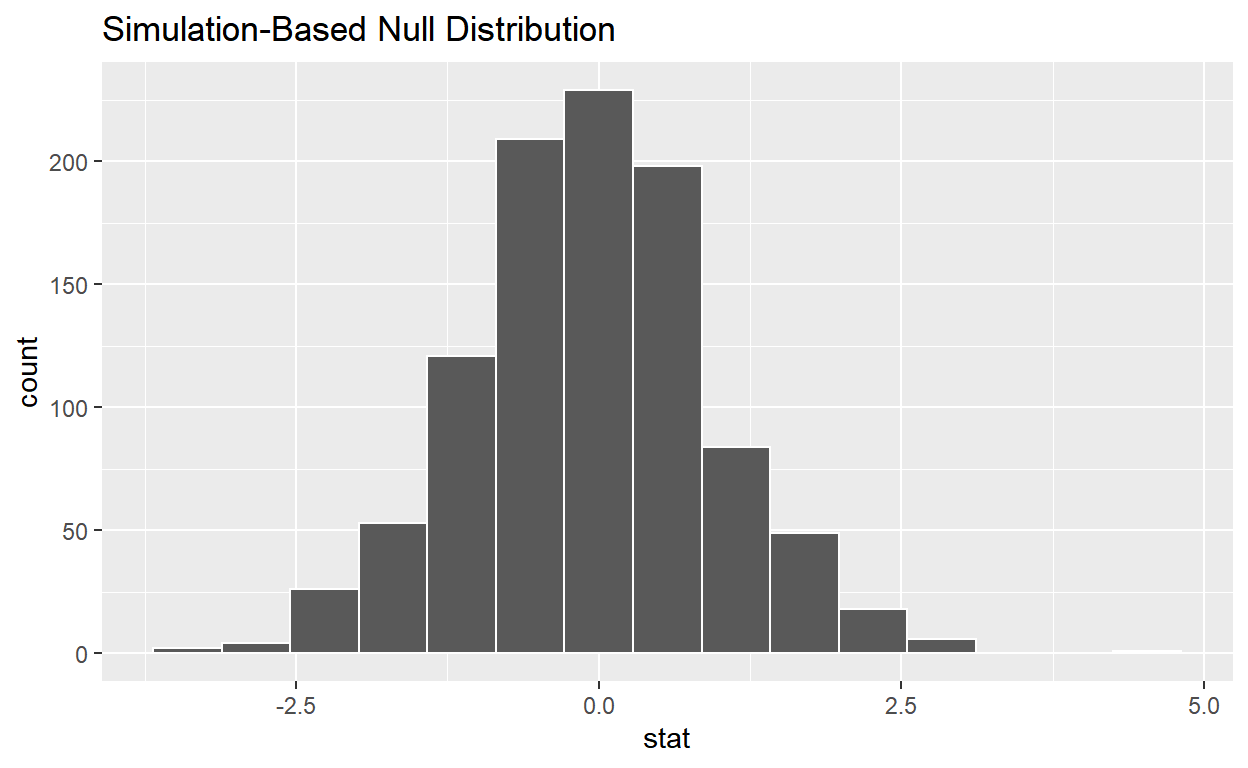• `calculate` the statistic from your observed data.

• Assign the output to `observed_t_2_sample_stat`

• Display `observed_t_2_sample_stat`

``````observed_t_2_sample_stat <- hr_2 %>%
specify(response = hours, explanatory = gender) %>%
calculate(stat = "t", order = c("female", "male"))

observed_t_2_sample_stat
``````
``````Response: hours (numeric)
Explanatory: gender (factor)
# A tibble: 1 x 1
stat
<dbl>
1  1.78``````

• `get_p_value` from your simulated null distribution and the observed statistic
``````null_t_distribution %>%
get_p_value(obs_stat = observed_t_2_sample_stat, direction = "two-sided")
``````
``````# A tibble: 1 x 1
p_value
<dbl>
1   0.086``````

• `shade_p_value` on the simulated null distribution.
``````null_t_distribution %>%
visualize() +
shade_p_value(obs_stat = observed_t_2_sample_stat, direction = "two-sided")
``````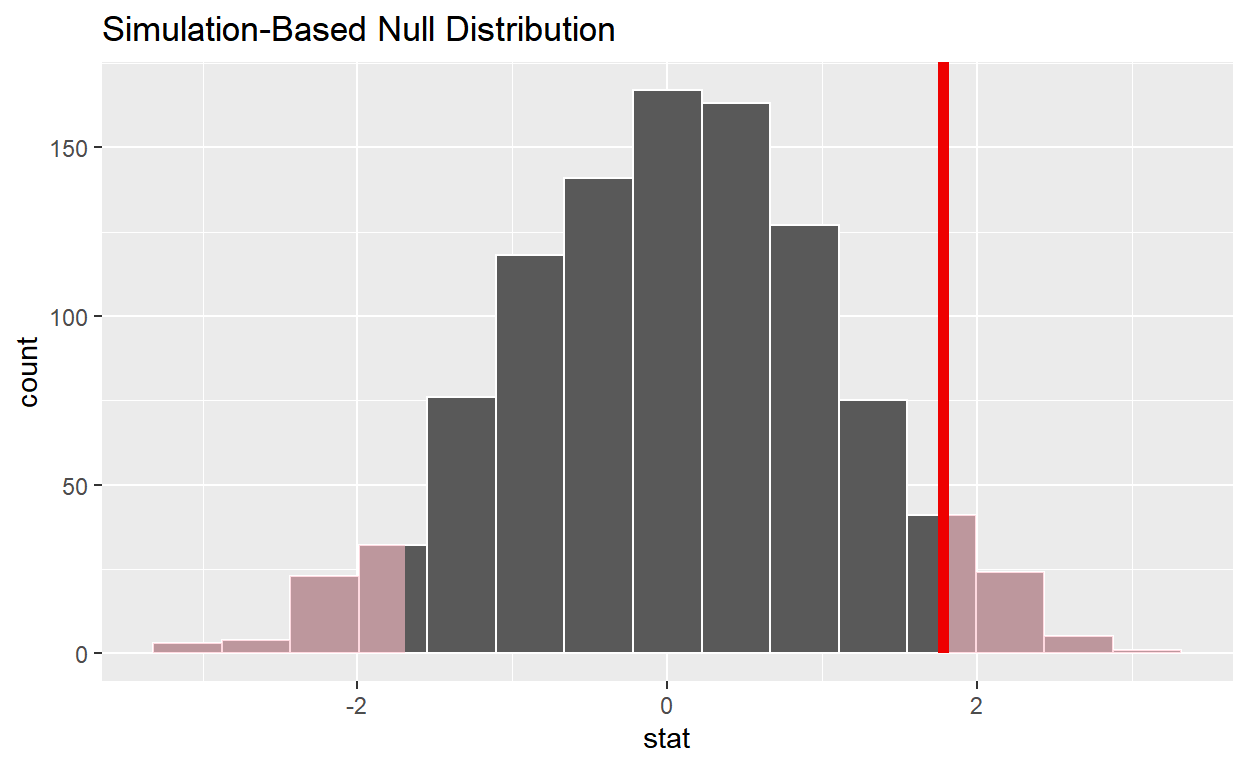• Is the p-value < 0.05? `no`

• Does your analysis support the null hypothesis that the true mean number of hours worked by female and male employees was the same? `yes`

# Question: ANOVA

• Load data into R, assign to `hr_anova`
``````hr_anova <- read_csv("https://estanny.com/static/week13/data/hr_2_tidy.csv",
col_types = "fddfff")
``````

Is the average number of hours worked the same for all three status (fired, ok, & promoted)?

• Use `skim` to summarize the data into `hr_anova` by `status`
``````hr_anova %>%
group_by(status) %>%
skim()
``````
 Name Piped data Number of rows 500 Number of columns 6 _______________________ Column type frequency: factor 3 numeric 2 ________________________ Group variables status

Variable type: factor

skim_variable status n_missing complete_rate ordered n_unique top_counts
gender promoted 0 1 FALSE 2 mal: 90, fem: 89
gender fired 0 1 FALSE 2 fem: 101, mal: 93
gender ok 0 1 FALSE 2 mal: 73, fem: 54
evaluation promoted 0 1 FALSE 4 goo: 70, ver: 62, fai: 24, bad: 23
evaluation fired 0 1 FALSE 4 bad: 78, fai: 72, goo: 25, ver: 19
evaluation ok 0 1 FALSE 4 bad: 53, fai: 46, ver: 15, goo: 13
salary promoted 0 1 FALSE 6 lev: 42, lev: 42, lev: 39, lev: 34
salary fired 0 1 FALSE 6 lev: 54, lev: 44, lev: 34, lev: 24
salary ok 0 1 FALSE 6 lev: 32, lev: 31, lev: 26, lev: 19

Variable type: numeric

skim_variable status n_missing complete_rate mean sd p0 p25 p50 p75 p100 hist
age promoted 0 1 40.63 11.25 20.4 30.75 41.10 50.25 59.9 ▆▇▇▇▇
age fired 0 1 40.03 11.53 20.3 29.45 40.40 50.08 59.9 ▇▅▇▆▆
age ok 0 1 38.50 11.98 20.3 28.15 38.70 49.45 59.9 ▇▆▅▅▆
hours promoted 0 1 59.21 12.66 35.0 49.75 58.90 70.65 79.9 ▅▆▇▇▇
hours fired 0 1 41.67 8.37 35.0 36.10 38.45 43.40 77.7 ▇▂▁▁▁
hours ok 0 1 47.35 10.86 35.0 37.10 45.70 54.50 78.9 ▇▅▃▂▁
• Employees that were fired worked an average of 41.7 hours per week.

• Employees that were ok worked an average of 47.4 hours per week.

• Employees that were promoted worked an average of 59.2 hours per week.

• Use `geom_boxplot` to plot distributions of hours worked by status.
``````hr_anova %>%
ggplot(aes(x = status, y = hours)) +
geom_boxplot()
``````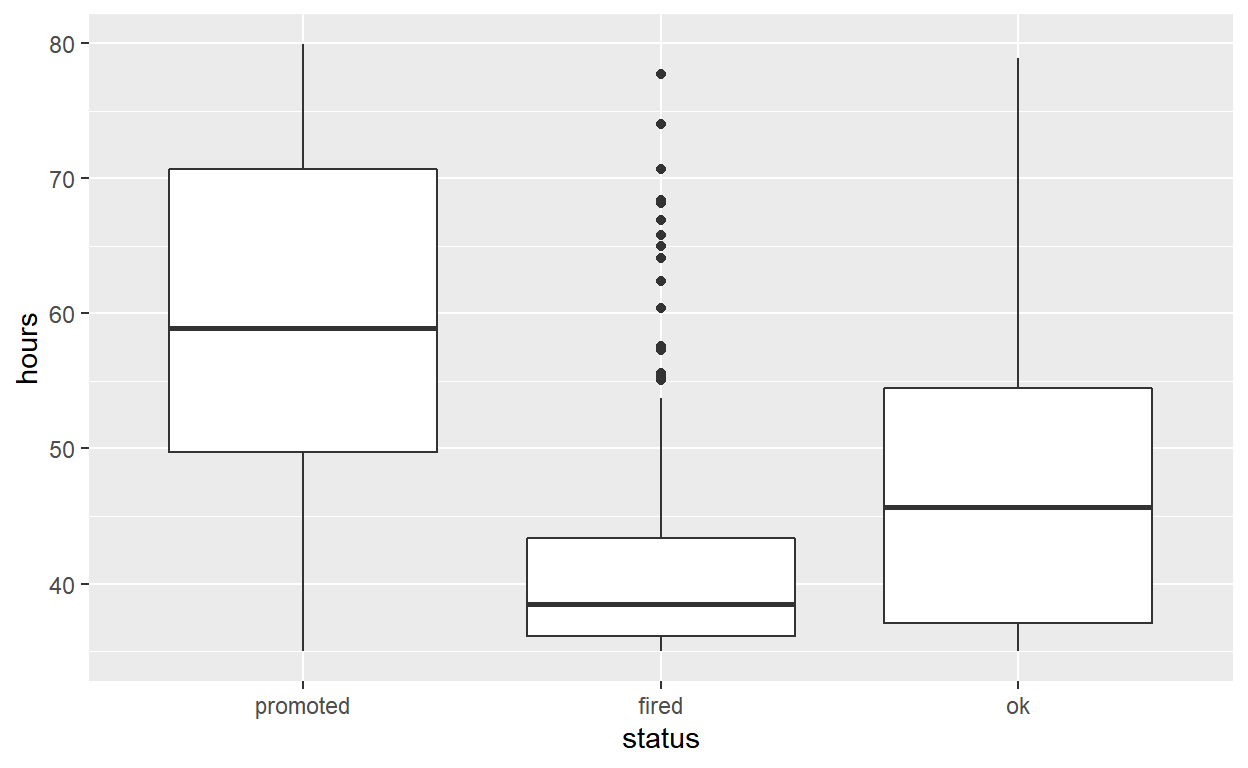• `specify` the variables of interest are `hours` and `status`
``````hr_anova %>%
specify(response = hours, explanatory = status)
``````
``````Response: hours (numeric)
Explanatory: status (factor)
# A tibble: 500 x 2
hours status
<dbl> <fct>
1  78.1 promoted
2  35.1 fired
3  36.9 fired
4  38.5 fired
5  36.1 fired
6  78.1 promoted
7  76   promoted
8  35.6 fired
9  35.6 ok
10  56.8 promoted
# ... with 490 more rows``````

• `hypothesize` that the number of hours worked and status are independent.
``````hr_anova %>%
specify(response = hours, explanatory = status) %>%
hypothesise(null = "independence")
``````
``````Response: hours (numeric)
Explanatory: status (factor)
Null Hypothesis: independence
# A tibble: 500 x 2
hours status
<dbl> <fct>
1  78.1 promoted
2  35.1 fired
3  36.9 fired
4  38.5 fired
5  36.1 fired
6  78.1 promoted
7  76   promoted
8  35.6 fired
9  35.6 ok
10  56.8 promoted
# ... with 490 more rows``````

• `generate` 1000 replicates representing the null hypothesis
``````hr_anova %>%
specify(response = hours, explanatory = status) %>%
hypothesise(null = "independence") %>%
generate(reps = 1000, type = "permute")
``````
``````Response: hours (numeric)
Explanatory: status (factor)
Null Hypothesis: independence
# A tibble: 500,000 x 3
# Groups:   replicate [1,000]
hours status   replicate
<dbl> <fct>        <int>
1  41.9 promoted         1
2  36.7 fired            1
3  35   fired            1
4  58.9 fired            1
5  36.1 fired            1
6  39.4 promoted         1
7  54.3 promoted         1
8  59.2 fired            1
9  40.2 ok               1
10  35.3 promoted         1
# ... with 499,990 more rows``````
• The output has 500,000 rows.

• `calculate` the distribution of statistics from generated data

• Assign the output to `null_distribution_anova`

• Display `null_distribution_anova`

``````null_distribution_anova <- hr_anova %>%
specify(response = hours, explanatory = status) %>%
hypothesise(null = "independence") %>%
generate(reps = 1000, type = "permute") %>%
calculate(stat = "F")

null_distribution_anova
``````
``````Response: hours (numeric)
Explanatory: status (factor)
Null Hypothesis: independence
# A tibble: 1,000 x 2
replicate  stat
<int> <dbl>
1         1 0.312
2         2 2.85
3         3 0.369
4         4 0.142
5         5 0.511
6         6 2.73
7         7 1.06
8         8 0.171
9         9 0.310
10        10 1.11
# ... with 990 more rows``````
• `null_distribution_anova` has 1000 F-stats.

• `visualize` the statistic from your observed data.
``````visualise(null_distribution_anova)
``````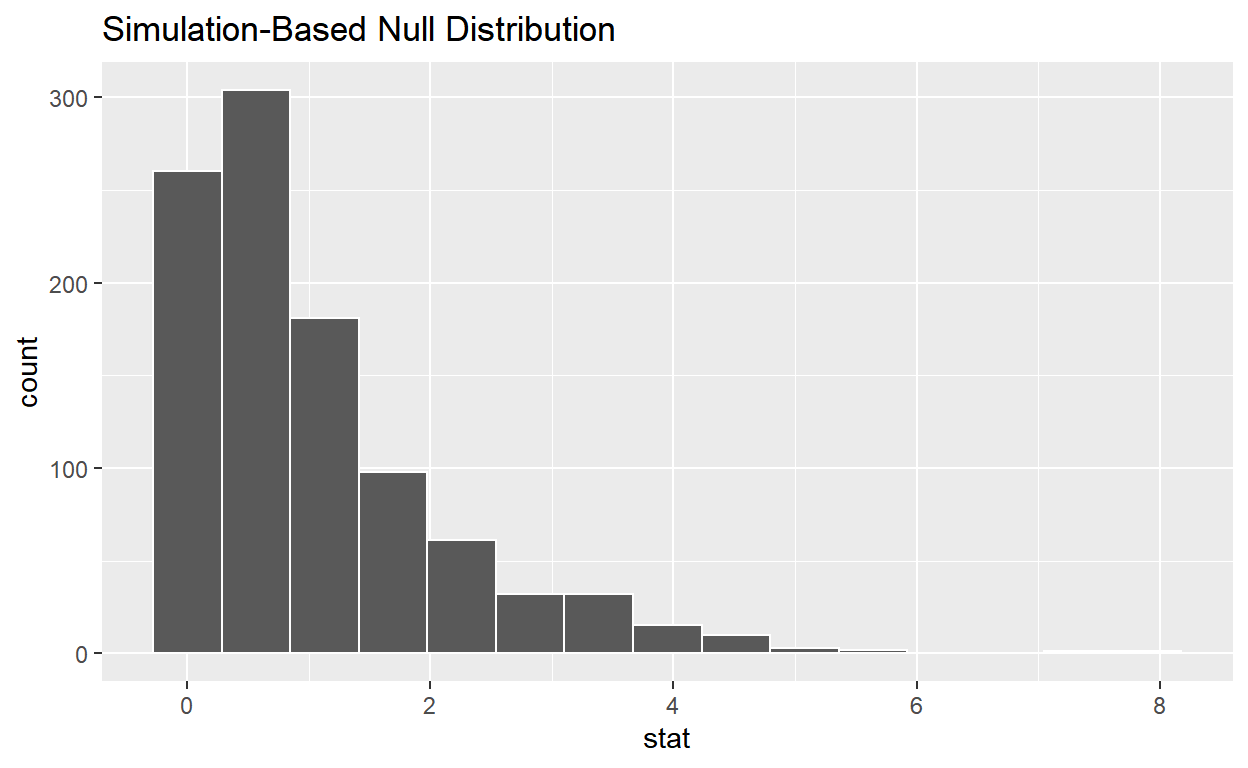• `calculate` the statistic from your observed data.

• Assign the output to `observed_f_sample_stat`

• Display `observed_f_sample_stat`

``````observed_f_sample_stat <- hr_anova %>%
specify(response = hours, explanatory = status) %>%
calculate(stat = "F")

observed_f_sample_stat
``````
``````Response: hours (numeric)
Explanatory: status (factor)
# A tibble: 1 x 1
stat
<dbl>
1  128.``````

• `get_p_value` from the simulated null distribution and the observed statistic.
``````null_distribution_anova %>%
get_p_value(obs_stat = observed_f_sample_stat, direction = "greater")
``````
``````# A tibble: 1 x 1
p_value
<dbl>
1       0``````

• `shade_p_value` on the simulated null distribution.
``````null_t_distribution %>%
visualize() +
shade_p_value(obs_stat = observed_f_sample_stat, direction = "greater")
``````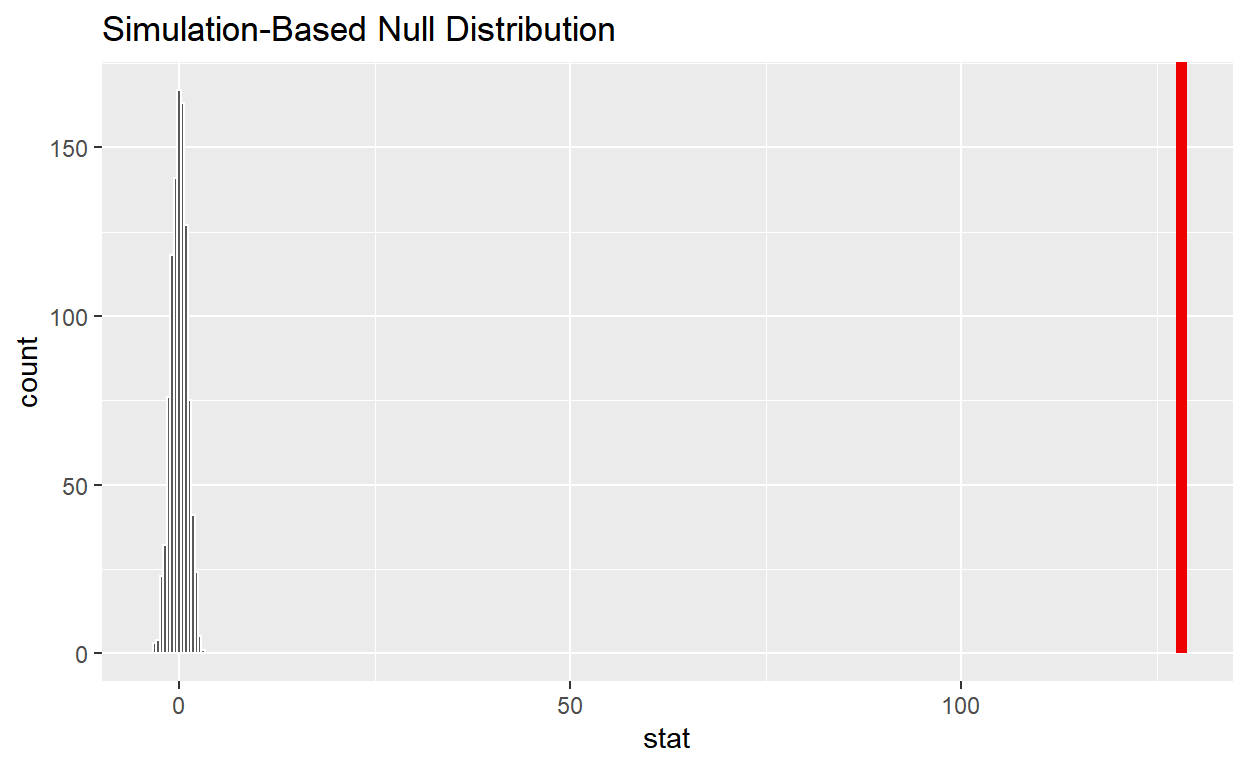``````ggsave(filename = "preview.png", path = here::here("_posts", "2022-04-29-hypothesis-testing"))
``````

• Is the p-value < 0.05? `yes`

• Does your analysis support the null hypothesis that the true means of the number of hours worked for those that were “fired”, “ok” and “promoted” were the same? `no`